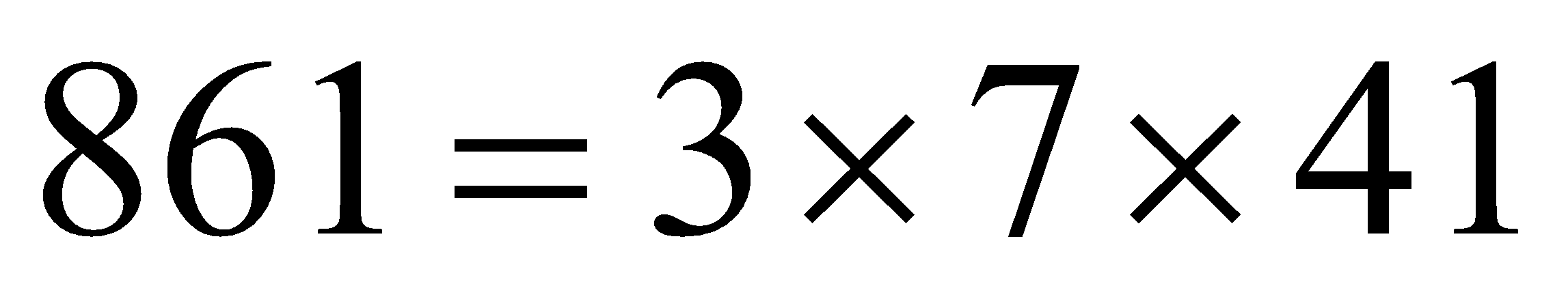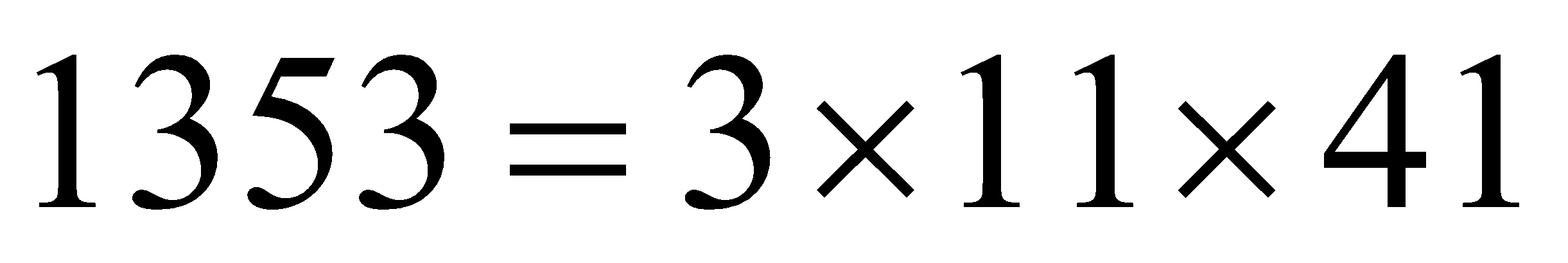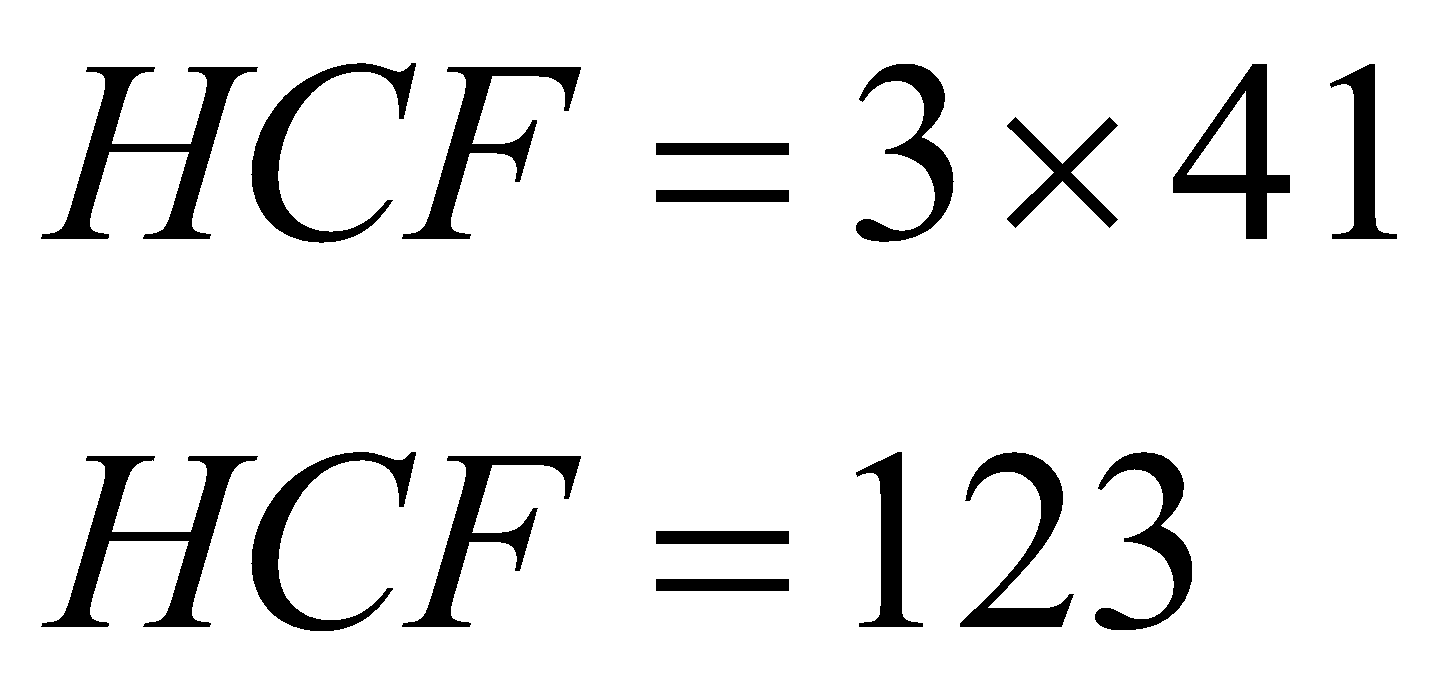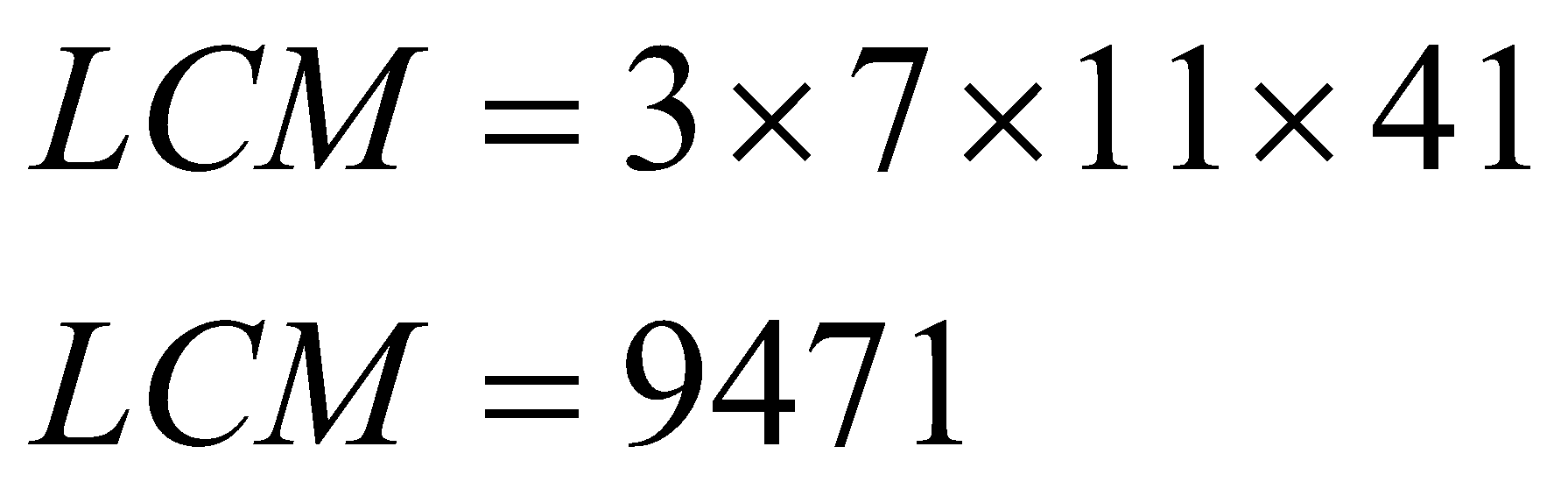Find the HCF and LCM of 861, 1353.

# Find the HCF and LCM of 861, 1353.

1. A
33, 3157
2. B
123, 9471
3. C
123, 3157
4. D
33, 9471

Fill Out the Form for Expert Academic Guidance!l

+91

Live ClassesBooksTest SeriesSelf Learning

Verify OTP Code (required)

### Solution:

Concept- In order to get the LCM and HCF we must first know their factors.
We can get the factors by prime factorisation method.
With prime factorisation we can get the prime factors of that number.
Factors of 861:Factors of 1353:HCF of 861 and 1353 is:
The common factors of both are 3 and 41.LCM of 861 and 1353 is:Hence, the correct answer is option 2.

## Related content

 Area of Square Area of Isosceles Triangle Pythagoras Theorem Triangle Formula Perimeter of Triangle Formula Area Formulae Volume of Cone Formula Matrices and Determinants_mathematics Critical Points Solved Examples Type of relations_mathematics+91

Live ClassesBooksTest SeriesSelf Learning

Verify OTP Code (required)